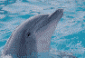# likelihood function for type=cov/cor

4 posts / 0 newOffline
Joined: 10/19/2009 - 15:38
likelihood function for type=cov/cor

the default likelihood function for observed data of type=cov or cor multiplies
log(det(predictedCov)) + trace(observedCov %*% inverse(predictedCov))
by (numObs - 1). my understanding is that both the derivations from the multivariate normal pdf and the wishart pdf lead to this being multiplied by numObs and not (numObs - 1). (note that math/stat treatment of the wishart talks of "degrees of freedom" but the actual algebra defines this quantity as numObs).

i stand to be corrected here.
greg

PS for most stuff in SEM, this is completely trivial. i am, however, in the process of writing an efficient algorithm for pedigree analysis in openmx (the genetic stuff) and want to know if i do or do not need to correct the likelihood function. also, if i am correct, the MLE will not be correct for the pedigree analysis.Offline
Joined: 07/31/2009 - 15:12
Interesting. I would agree,

Interesting. I would agree, though the use of n vs n-1 for the calculation of the data covariance matrix likely have some effect as well.

In the case of minimization, the choice of either n or n-1 shouldn't make a difference, as they amount to a linear transformation of the parameter space that won't affect where the minima are. It will affect absolute criteria for convergence, however. I don't see how changing from n-1 to n will affect the MLE.

This issue got me thinking about a problem equating the -2LLs of covariance and FIML models. I couldn't solve a few months ago, and your note helped me solve it (thanks! see thread http://openmx.psyc.virginia.edu/thread/806). Based solely on that, n seems to be superior to n-1.Offline
Joined: 10/19/2009 - 15:38
ryne, you are totally correct

ryne,
you are totally correct when as i stated "for most stuff in SEM, this is completely trivial." for a quick and dirty bottom line, skip the following and look at the last paragraph.

a pedigree analysis, however, will not always conform to the "most stuff in SEM." consider an R data.frame that has a family as a row with a maximum of 2 offspring per family. when a family has two offspring, the observation consists of fathers variables, then mother's variables, then offspring 1's variables, then offspring 2's variables. if the family, however, had only 1 offspring, then all of offspring 2's variables will be NA.

one could easily deal with this by creating an MxData object of type="raw." when there are a large number of pedigrees with a large number of variables per individual and missing values in variables, however, this strategy may be very inefficient.

a efficient strategy is to organize the data set according to the pattern of NAs. for any row with a unique pattern of NAs, add it to a data.frame that will eventually become an MxData object of type="raw." for those rows with the same pattern of NAs, compute the covariance matrix and the vector of means. this set becomes a separate MxData object of type="cov".

if (1) the user computed the covariance matrix with the divisor N and not (N - 1) and (2) OpenMx used the correct definitions of the wishart, then one can validly add the log likelihoods from the type="cov" MxData objects to the log likelihood of the type="raw" MxModel. as it stands, even if the user did (1), the current OpenMx calculation of the log likelihood for a type="cov" MxData object will result in an incorrect log likelihood for the model.

also--and much more salient--if there is a correct and incorrect likelihood function for the wishart, should not OpenMx implement the correct one?

gregOffline
Joined: 07/31/2009 - 15:12
greg, Thanks for the thorough

greg,

Thanks for the thorough response. I'm in very close agreement with you, and like your comments, my pressing questions can be found at the end.

Your description points to one of my motivations for discovering the ML-FIML relationships: providing speed-ups for FIML by splitting data into subsets with identical patterns of missingness/definition variables. I didn't realize that ML was using n-1 instead of n (which I wouldn't have to admit if we weren't open source), so I spent time looking through FIML for problems and correcting the input covariance matrix but never looked at the numObs parameter in the ML objective.

The case you describe is one of a more general class of problems that can be solved more efficiently with a multiple-group approach to the FIML problem. Long-term, I/we hope to implement this type of speed-up into FIML. Short-term, you are correct that you can implement your own version of this speed-up by splitting your data into identical patterns of missingness and run ML objectives, provided you correctly weight the objective functions to account for the sampling corrections.

I agree with you that OpenMx should be correct in all of its calculations, as well as open about that those calculations are so that conversations like these can occur. I agree with you regarding using N rather than N-1 based on my understanding of the ML likelihood function (which comes from Loehlin's text), but I'm unsure of how using N rather than N-1 in the data covariance calculation yields a correct ML estimate. If the data are biased estimates of the population parameters when the sampling correction is disregarded, how can the model fit to those data be proper MLEs?

As always, thanks for contributing to the project.

ryne